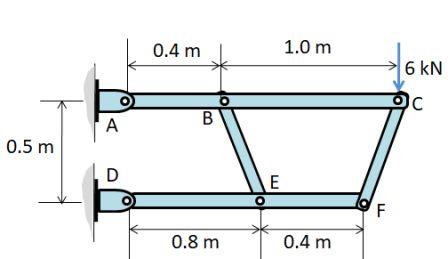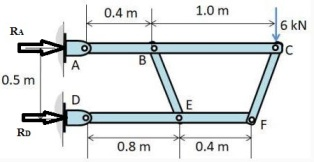# The form supports a 6-kN vertical load at ''C''. The bars ''ABC'' and 'DEF'' are horizontal....

## Question:

The form supports a 6-kN vertical load at C. The bars ABC and 'DEF are horizontal.

Determine the reaction on the frame at A and D.## Truss :

A truss is a combination of elements or members to develop a bigger structure that can sustain the desirable external load .

Example- a roof, a bridge , etc .It must be designed for suitable life and therefore of suitable material.

## Answer and Explanation:

Become a Study.com member to unlock this answer! Create your account

Consider the static equilibrum of the whole frame.{eq}\sum H = 0 \\ R_{A}+R_{D}=0 \\ \sum M_{A}=0 \\ (R_{D}\times 0.5)-(6\times 1.4)=0...

See full answer below.

#### Learn more about this topic:External Force: Definition & Examples

from

Chapter 1 / Lesson 22
32K

In this lesson, we are going to learn about external forces and their effects on the body to which they are applied. We are also going to distinguish external forces from internal forces.